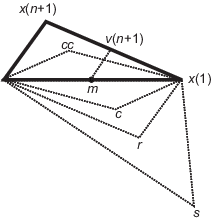## `fminsearch` Algorithm

`fminsearch` uses the Nelder-Mead simplex algorithm as described in Lagarias et al. . This algorithm uses a simplex of n + 1 points for n-dimensional vectors x. The algorithm first makes a simplex around the initial guess x0 by adding 5% of each component x0(i) to x0, and using these n vectors as elements of the simplex in addition to x0. (The algorithm uses 0.00025 as component i if x0(i) = 0.) Then, the algorithm modifies the simplex repeatedly according to the following procedure.

Note

The keywords for the `fminsearch` iterative display appear in bold after the description of the step.

1. Let x(i) denote the list of points in the current simplex, i = 1,...,n + 1.

2. Order the points in the simplex from lowest function value f(x(1)) to highest f(x(n + 1)). At each step in the iteration, the algorithm discards the current worst point x(n + 1), and accepts another point into the simplex. [Or, in the case of step 7 below, it changes all n points with values above f(x(1))].

3. Generate the reflected point

r = 2mx(n + 1),

where

m = Σx(i)/n, i = 1...n,

and calculate f(r).

4. If f(x(1)) ≤ f(r) < f(x(n)), accept r and terminate this iteration. Reflect

5. If f(r) < f(x(1)), calculate the expansion point s

s = m + 2(mx(n + 1)),

and calculate f(s).

1. If f(s) < f(r), accept s and terminate the iteration. Expand

2. Otherwise, accept r and terminate the iteration. Reflect

6. If f(r) ≥ f(x(n)), perform a contraction between m and eitherx(n + 1) or r, depending on which has the lower objective function value.

1. If f(r) < f(x(n + 1)) (that is, r is better than x(n + 1)), calculate

c = m + (rm)/2

and calculate f(c). If f(c) < f(r), accept c and terminate the iteration. Contract outside

Otherwise, continue with Step 7 (Shrink).

2. If f(r) ≥ f(x(n + 1)), calculate

cc = m + (x(n + 1) – m)/2

and calculate f(cc). If f(cc) < f(x(n + 1)), accept cc and terminate the iteration. Contract inside

Otherwise, continue with Step 7 (Shrink).

7. Calculate the n points

v(i) = x(1) + (x(i) – x(1))/2

and calculate f(v(i)), i = 2,...,n + 1. The simplex at the next iteration is x(1), v(2),...,v(n + 1). Shrink

The following figure shows the points that `fminsearch` might calculate in the procedure, along with each possible new simplex. The original simplex has a bold outline. The iterations proceed until they meet a stopping criterion.